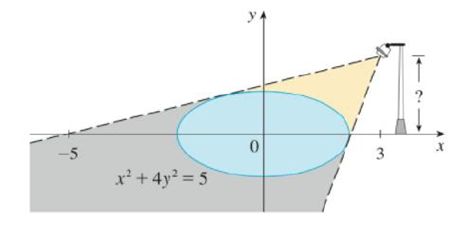Chapter 3.5, Problem 80E

Chapter
Section
Textbook Problem

The figure shows a lamp located three units to the right of the y-axis and a shadow created by the elliptical region x2 + 4y2 ≤ 5. If the point (–5. 0) is on the edge of the shadow, how far above the x-axis is the lamp located?To determine

To find: How far above x-axis is the lamp located.

Explanation

Given:

The lamp located three unit from the right of y axis,

The shadow created by elliptical region x2+4y25.

The point (5,0) is on the edge of the shadow.

Calculation:

Let h be the height of the lamp.

Let (a,b) be an point of the tangency through the point (3,h) and (5,0).

The slope of the line passing through the point (3,h) and (5,0),

m=0h53=h8

Thus, the slope of the tangent at (a,b) is m=h8 (1)

Consider the equation of the ellipse x2+4y2=5,

Differentiate with respect to x,

ddx(x2)+4ddx(y2)=ddx(5)2x+4(2ydydx)=0dydx=2x8ydydx=x4y

That is, the slope of the tangent to the ellipse is, dydx=x4y

Still sussing out bartleby?

Check out a sample textbook solution.

See a sample solution

The Solution to Your Study Problems

Bartleby provides explanations to thousands of textbook problems written by our experts, many with advanced degrees!

Get Started

Find the inverse function of f(x)=x+12x+1.

Single Variable Calculus: Early Transcendentals, Volume I

210 Find ab. a=1.5,0.4, b=4,6

Calculus (MindTap Course List)

True or False: is a convergent series.

Study Guide for Stewart's Single Variable Calculus: Early Transcendentals, 8th